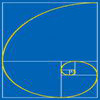# Resources tagged with: Golden ratio

Filter by: Content type:
Age range:
Challenge level:

### There are 16 results

Broad Topics > Fractions, Decimals, Percentages, Ratio and Proportion > Golden ratio### Golden Fractions

##### Age 16 to 18Challenge Level

Find the link between a sequence of continued fractions and the ratio of succesive Fibonacci numbers.### Golden Powers

##### Age 16 to 18Challenge Level

You add 1 to the golden ratio to get its square. How do you find higher powers?### Golden Fibs

##### Age 16 to 18Challenge Level

When is a Fibonacci sequence also a geometric sequence? When the ratio of successive terms is the golden ratio!### Pythagorean Golden Means

##### Age 16 to 18Challenge Level

Show that the arithmetic mean, geometric mean and harmonic mean of a and b can be the lengths of the sides of a right-angles triangle if and only if a = bx^3, where x is the Golden Ratio.### The Golden Ratio, Fibonacci Numbers and Continued Fractions.

##### Age 14 to 16

An iterative method for finding the value of the Golden Ratio with explanations of how this involves the ratios of Fibonacci numbers and continued fractions.### Pentakite

##### Age 14 to 18Challenge Level

ABCDE is a regular pentagon of side length one unit. BC produced meets ED produced at F. Show that triangle CDF is congruent to triangle EDB. Find the length of BE.### Golden Ratio

##### Age 16 to 18Challenge Level

Solve an equation involving the Golden Ratio phi where the unknown occurs as a power of phi.### Leonardo of Pisa and the Golden Rectangle

##### Age 7 to 16

Leonardo who?! Well, Leonardo is better known as Fibonacci and this article will tell you some of fascinating things about his famous sequence.### Pent

##### Age 14 to 18Challenge Level

The diagram shows a regular pentagon with sides of unit length. Find all the angles in the diagram. Prove that the quadrilateral shown in red is a rhombus.### Golden Thoughts

##### Age 14 to 16Challenge Level

Rectangle PQRS has X and Y on the edges. Triangles PQY, YRX and XSP have equal areas. Prove X and Y divide the sides of PQRS in the golden ratio.### Golden Triangle

##### Age 16 to 18Challenge Level

Three triangles ABC, CBD and ABD (where D is a point on AC) are all isosceles. Find all the angles. Prove that the ratio of AB to BC is equal to the golden ratio.### Whirling Fibonacci Squares

##### Age 11 to 16

Draw whirling squares and see how Fibonacci sequences and golden rectangles are connected.##### Age 16 to 18

What is the relationship between the arithmetic, geometric and harmonic means of two numbers, the sides of a right angled triangle and the Golden Ratio?### Darts and Kites

##### Age 14 to 16Challenge Level

Explore the geometry of these dart and kite shapes!### Gold Yet Again

##### Age 16 to 18Challenge Level

Nick Lord says "This problem encapsulates for me the best features of the NRICH collection."### Gold Again

##### Age 16 to 18Challenge Level

Without using a calculator, computer or tables find the exact values of cos36cos72 and also cos36 - cos72.